# 阅读记录How to Create a Custom 2D Physics Engine - Rangy Gaul（2）

## Friction, Scene and Jump Table

### Friction

once the objects land on the solid platform, they just sort of all press away and drift off the edges of the screen. This is due to a lack of friction simulation.一旦物体落在光滑的平台上，它们就会被压走，并从屏幕边缘漂移。这是由于缺乏摩擦模拟。

2d下，直接像处理普通冲量那样，然后把n换为t

$j = \frac{-(1 + e)((V^B - V^A) \cdot t)}{\frac{1}{mass^A} + \frac{1}{mass^B}}$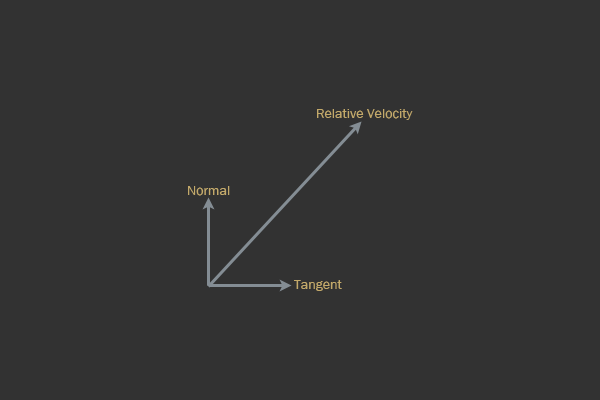Vectors of various types within the timeframe of a collision of rigid bodies.

$V^R = V^B-V^A \\ t = V^R- (V^R \cdot n)*n$

// Re-calculate relative velocity after normal impulse
// is applied (impulse from first article, this code comes
// directly thereafter in the same resolve function)
Vec2 rv = VB - VA

// Solve for the tangent vector
Vec2 tangent = rv - Dot( rv, normal ) * normal
t = tangent.Normalize( )

// Solve for magnitude to apply along the friction vector
float jt = -Dot( rv, t ) //我认为t就是tangent标准化后的
jt = jt / (1 / MassA + 1 / MassB) //这里简化了，没有-（1+e）


( 这段推导看的很迷惑)

### Coulomb's Law库伦定律

$F_f <= \mu F_n$

// Re-calculate relative velocity after normal impulse
// is applied (impulse from first article, this code comes
// directly thereafter in the same resolve function)
Vec2 rv = VB - VA

// Solve for the tangent vector
Vec2 tangent = rv - Dot( rv, normal ) * normal
t = tangent.Normalize( )

// Solve for magnitude to apply along the friction vector
float jt = -Dot( rv, t )
jt = jt / (1 / MassA + 1 / MassB)

// PythagoreanSolve = A^2 + B^2 = C^2, solving for C given A and B
// Use to approximate mu given friction coefficients of each body
float mu = PythagoreanSolve( A->staticFriction, B->staticFriction )

// Clamp magnitude of friction and create impulse vector
Vec2 frictionImpulse
if(abs( jt ) < j * mu)
frictionImpulse = jt * t
else
{
dynamicFriction = PythagoreanSolve( A->dynamicFriction, B->dynamicFriction )
frictionImpulse = -j * t * dynamicFriction
}

// Apply
A->velocity -= (1 / A->mass) * frictionImpulse
B->velocity += (1 / B->mass) * frictionImpulse


y有些摩擦系数的求解：

$Friction = \sqrt{Friction_A^2+Friction_B^2}$

### 静摩擦和动摩擦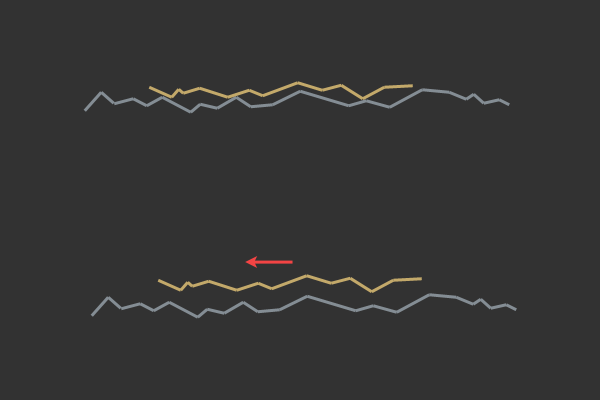Microscopic view of what causes energy of activation due to friction.

### Scene

Scene类充当包含物理模拟场景的所有内容的容器。它调用并使用任何广义阶段的结果，包含所有刚体，运行碰撞检查并调用分辨率。它还集成了所有活动对象。场景还会与用户交互(就像程序员使用物理引擎一样)。

class Scene
{
public:
Scene( Vec2 gravity, real dt );
~Scene( );

void SetGravity( Vec2 gravity )
void SetDT( real dt )

Body *CreateBody( ShapeInterface *shape, BodyDef def )

// Inserts a body into the scene and initializes the body (computes mass).
//void InsertBody( Body *body )

// Deletes a body from the scene
void RemoveBody( Body *body )

// Updates the scene with a single timestep
void Step( void )

float GetDT( void )
Vec2 GetGravity( void )
void QueryAABB( CallBackQuery cb, const AABB& aabb )
void QueryPoint( CallBackQuery cb, const Point2& point )

private:
float dt     // Timestep in seconds
float inv_dt // Inverse timestep in sceonds
uint32 body_count
Vec2 gravity
bool debug_draw
};


Scene类没有什么特别复杂的地方。这样做的目的是让用户能够轻松地添加和移除刚体。BodyDef是一种包含刚体所有信息的结构（类似UE4的bodySetup），可用于允许用户将值作为一种配置结构插入。

### JumpTable 碰撞查询跳转表

c++中，我知道有两种主要的方法:双重分派和2D跳转表。在我自己的测试中，我发现了2D跳转表更好用，所以我将详细介绍如何实现它。如果你计划使用C或c++以外的其他语言我相信可以构造一个函数或函子对象数组类似于一个函数指针表(这是另一个原因我选择了谈论跳表而不是其他选项更特定于c++)。

enum Animal
{
Rabbit
Duck
Lion
};

const void (*talk)( void )[] = {
RabbitTalk,
DuckTalk,
LionTalk,
};

// Call a function from the table with 1D virtual dispatch
talk[Rabbit]( ) // calls the RabbitTalk function


collisionCallbackArray = {
AABBvsAABB
AABBvsCircle
CirclevsAABB
CirclevsCircle
}

// Call a collsion routine for collision detection between A and B
// two colliders without knowing their exact collider type
// type can be of either AABB or Circle
collisionCallbackArray[A->type][B->type]( A, B )


## Oriented Rigid Bodies

### Cross Product叉积

// Two crossed vectors return a scalar
float CrossProduct( const Vec2& a, const Vec2& b )
{
return a.x * b.y - a.y * b.x;
}

// More exotic (but necessary) forms of the cross product
// with a vector a and scalar s, both returning a vector
Vec2 CrossProduct( const Vec2& a, float s )
{
return Vec2( s * a.y, -s * a.x );
}

Vec2 CrossProduct( float s, const Vec2& a )
{
return Vec2( -s * a.y, s * a.x );
}


### 转矩和角速度### 积分

struct RigidBody
{
Shape *shape

// Linear components
Vec2 position
Vec2 velocity
float acceleration

// Angular components
float angularVelocity
float torque
};


const Vec2 gravity( 0, -10.0f )
velocity += (force * 1.0f / mass) + gravity * dt
angularVelocity += torque * (1.0f / momentOfInertia) * dt
position += velocity * dt
orient += angularVelocity * dt


struct Mat22
{
union
{
struct
{
float m00, m01
float m10, m11;
};

struct
{
Vec2 xCol;
Vec2 yCol;
};
};
};


2x2的矩阵类

Mat22 m( PI / 2.0f );
Vec2 r = m.ColX( ); // retrieve the x axis column


Mat22::Mat22( const Vec2& x, const Vec2& y )
{
m00 = x.x;
m01 = x.y;
m01 = y.x;
m11 = y.y;
}

// or

Mat22::Mat22( const Vec2& x, const Vec2& y )
{
xCol = x;
yCol = y;
}


Mat2( real radians )
{
real c = std::cos( radians );
real s = std::sin( radians );

m00 = c; m01 = -s;
m10 = s; m11 =  c;
}

// Rotate a vector
const Vec2 operator*( const Vec2& rhs ) const
{
return Vec2( m00 * rhs.x + m01 * rhs.y, m10 * rhs.x + m11 * rhs.y );
}


### Transforming to a Basis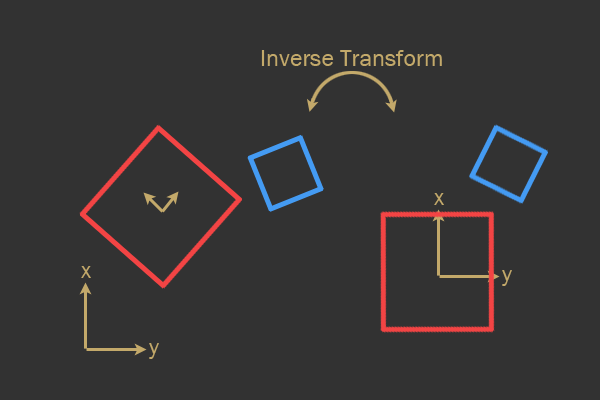Inverse transformation (left to right) from world space to model space of the red polygon.

### Collision Detection and Manifold Generation

However, instead of projecting each polygon's extents onto each other, there is a slightly newer and more efficient method, as outlined by Dirk Gregorius in his 2013 GDC Lecture (slides available here for free).

#### 支撑点

Support Point 支撑点的概念很重要

// The extreme point along a direction within a polygon
Vec2 GetSupport( const Vec2& dir )
{
real bestProjection = -FLT_MAX;
Vec2 bestVertex;

for(uint32 i = 0; i < m_vertexCount; ++i)
{
Vec2 v = m_vertices[i];
real projection = Dot( v, dir );

if(projection > bestProjection)
{
bestVertex = v;
bestProjection = projection;
}
}

return bestVertex;
}


#### 寻找分离轴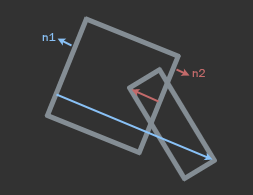real FindAxisLeastPenetration( uint32 *faceIndex, PolygonShape *A, PolygonShape *B )
{
real bestDistance = -FLT_MAX;
uint32 bestIndex;

for(uint32 i = 0; i < A->m_vertexCount; ++i)
{
// Retrieve a face normal from A
Vec2 n = A->m_normals[i];

// Retrieve support point from B along -n
Vec2 s = B->GetSupport( -n );

// Retrieve vertex on face from A, transform into
// B's model space
Vec2 v = A->m_vertices[i];

// Compute penetration distance (in B's model space)
real d = Dot( n, s - v );

// Store greatest distance
if(d > bestDistance)
{
bestDistance = d;
bestIndex = i;
}
}

*faceIndex = bestIndex;
return bestDistance;
}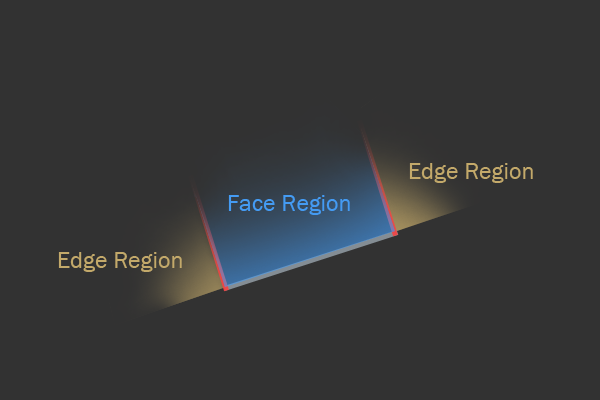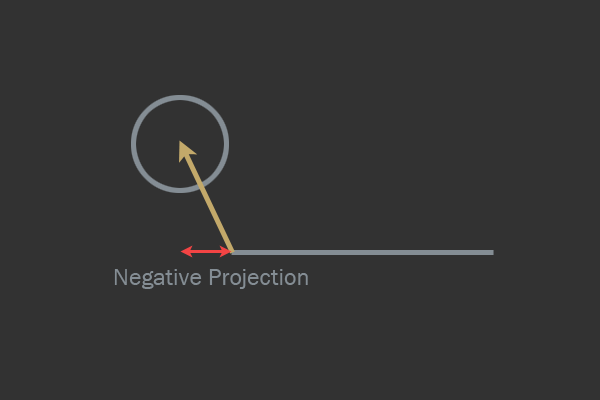### 碰撞解决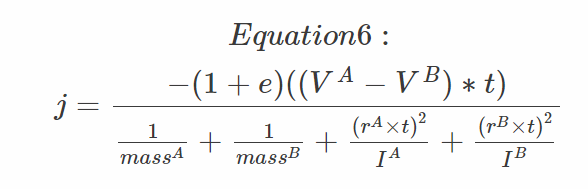A more in-depth derivation of this equation can be found on Chris Hecker's site.关于这个方程的更深入的推导可以在Chris Hecker的网站上找到。

r是一个从物体的质点到接触点的向量

$V' = V + \omega \cross r$

void Body::ApplyImpulse( const Vec2& impulse, const Vec2& contactVector )
{
velocity += 1.0f / mass * impulse;
angularVelocity += 1.0f / inertia * Cross( contactVector, impulse );
}


contactVector是什么方向的，质点到接触点的向量

## 引用

https://gamedevelopment.tutsplus.com/tutorials/how-to-create-a-custom-2d-physics-engine-friction-scene-and-jump-table--gamedev-7756

https://gamedevelopment.tutsplus.com/tutorials/how-to-create-a-custom-2d-physics-engine-oriented-rigid-bodies--gamedev-8032

posted @ 2021-09-06 01:13  飞翔的子明  阅读(206)  评论(0编辑  收藏  举报